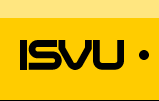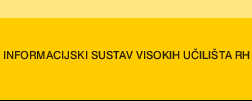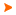Podaci o VU Početna stranicaArhiva nastavnih programaArhiva ak. kalendaraMathematics IX
 Abbreviation: MAT IX Load: 15(L) + 15(E) + 0(LE) + 0(CE) + 0(PEE) + 0(FE) + 0(S) + 0(DE) + 0(P) + 0(FLE) + 0()
 Lecturers in charge: prof. dr. sc. Sanja Singer Lecturers: Neven Krajina ( Exercises ) prof. dr. sc. Sanja Singer ( Exercises ) Saša Stanko ( Exercises ) Course description: Course objectives: To understand concepts of the numerical mathematics and scientific computing. Usage of the numerical methods with emphasys on possible errors in obtained results.Enrolment requirements and required entry competences for the course: Student responsibilities: Grading and evaluation of student work over the course of instruction and at a final exam: Passing two midterm exams (45%) is equivalent to a written exam.Methods of monitoring quality that ensure acquisition of exit competences: Written exam.Upon successful completion of the course, students will be able to (learning outcomes): After the final exam, students will be able to describe the basics of the numerical methods, and find the solustion the simple numerical problems. Especially, they will be able to describe errors of the computer arithmetics.Lectures 1. Introduction to numerical mathematics and scientific computing.2. IEEE arithmetics. Errors.3. Direct methods for solution of linear systems. Gaussian eliminations.4. LR factorization. Pivoting. Condition number.5. Iterative methods for solution of linear systems. Convergence.6. Jacobi, GaussSeidel and SOR methods. Convergence.7. Examples of the usage of the iterative methods for linear systems.8. Introduction to approximations. Types of approximations.9. Polynomial interpolation.10. Spline interpolation.11. Method of least squares.12. Numerical integration. NewtonCotes i Gaussian formulae.13. Trapezoidal and Simpson"s rule. Robmerg"s algorithm.14. Methods for the initial value problem ODE. RK methods and multistep methods.15. Stiff differential equations.Exercises 1. Introduction to numerical mathematics and scientific computing.2. IEEE arithmetics. Errors.3. Direct methods for solution of linear systems. Gaussian eliminations.4. LR factorization. Pivoting. Condition number.5. Iterative methods for solution of linear systems. Convergence.6. Jacobi, GaussSeidel and SOR methods. Convergence.7. Examples of the usage of the iterative methods for linear systems.8. Introduction to approximations. Types of approximations.9. Polynomial interpolation.10. Spline interpolation.11. Method of least squares.12. Numerical integration. NewtonCotes i Gaussian formulae.13. Trapezoidal and Simpson"s rule. Robmerg"s algorithm.14. Methods for the initial value problem ODE. RK methods and multistep methods.15. Stiff differential equations.
 Lecture languages: hr
 Compulsory literature: 1. Pisani materijali za predmet Matematika 9, dostupni na e-ucenje.fsb.hr.
 Recommended literature: 2. Ivo Alfirević i dr., Inženjerski priručnik 1, Školska knjiga, Zagreb, 1996.Legend L - Lectures FLE - Practical foreign language exercises - E - Exercises LE - Laboratory exercises CE - Project laboratory PEE - Physical education excercises FE - Field exercises S - Seminar DE - Design exercises P - Practicum * - Not graded| Početna stranica | Visoka učilišta | Korisničke stranice | | Na vrh | Copyright (c) 2006. Ministarstva znanosti, obrazovanja i športa. Sva prava zadržana. Programska podrška (c) 2006. Fakultet elektrotehnike i računarstva. Oblikovanje(c) 2006. Listopad Web Studio. Posljednja izmjena 2019-06-07